# A little fun with stacking bricks

Before starting this post, I'd like to give credit to Apolline Pepin, who helped me designing the stacking algorithm on a nice summer day in the Pyrénées. Thanks again!

In :
%matplotlib inline
import pylab as pl
from IPython.html.widgets import interact


In this post, we're going to tackle the following problem:

Given a sequence of bricks, what is the best way to stack them on top of each other so as to cover the greatest possible horizontal distance?

By "stack them on top of each other", we mean two things:

• the bricks lie on top of each other (no glue or any kind of support)
• they're stable (gravity doesn't make the construction collapse)

This problem has some similarity with the Jenga game (see picture below), where players remove blocks of woods from an initially stable pyramid, until it collapses. The collapse happening to Jenga towers is essentially due to gravity: removing one block changes the static properties of the structure and destroys it.

In :
from IPython.display import Image
Image(url="http://kristinruffin.files.wordpress.com/2014/03/jenga-falling-for-web.jpg")

Out:Other examples of this sort of stacking exist. As an example we can cite the Nubian vault technique, whose key advantage Wikipedia says is the ability to be built "without any support or shuttering".

This post is comprised of several sections. We first describe our model and data representation of this problem in Python. We then move on to write some graphic functions before designing the meat of this post: the "stacking algorithm". Finally, we examine applications of our code.

## Modelling assumptions¶

In this post, we'll work with "bricks" that we will stack. Our modelling assumptions are as follows:

• a brick of width $w$ and height $h$ is modelled as tuple $(w, h)$
• a sequence of bricks to be stacked in a given order (the first on bottom, the last on top) is modelled as a list of bricks as defined above

Next, we're going to write a simple function that generates regular sequences of bricks, much like with Jenga, which will be used in the examples to follow. The function takes as arguments:

• the width and height of the brick sequence to be generated
• the number of bricks to generate
In :
def generate_brick_sequence(width, height, n):
return [(width, height) for i in range(n)]


Below, we test the output on an example case, generating a sequence of five bricks with width 3 and height 4:

In :
generate_brick_sequence(3, 4, 5)

Out:
[(3, 4), (3, 4), (3, 4), (3, 4), (3, 4)]

To continue, we will do two things, that will in time be translated to two functions.

• a way of plotting brick sequences
• a way to compute how to stack the bricks in a stable way for maximum range given a sequence of bricks as input

Among these two things, the first is easy. And fun. So we're starting with that.

## Plotting brick sequences¶

Below, we write a function that plots bricks using Rectangles, a functionality already provided by matplotlib.

The way it works is the following. We take inputs made of:

• a brick sequence
• the horizontal shifts to apply to each brick

And then we loop and draw each brick on top of each other, starting with the first one at the bottom and applying the horizontal shift given as an input. To make this function generic, we keep track of the total height, so as to stack bricks exactly above each other.

In :
from matplotlib.patches import Rectangle

def plot_brick_sequence(brick_sequence, shifts):
ax = pl.gca()
total_height = 0.
for brick, shift in zip(brick_sequence, shifts):
total_height += brick


We can immediately try our function on an exemple sequence (that I just made up: the stacking is NOT physcally acceptable).

In :
bricks = [(1, 2), (2, 3), (3, 4)]
shifts = [0, 0.5, 2]
plot_brick_sequence(bricks, shifts)
pl.xlim(0, 10)
pl.ylim(0, 10)

Out:
(0, 10)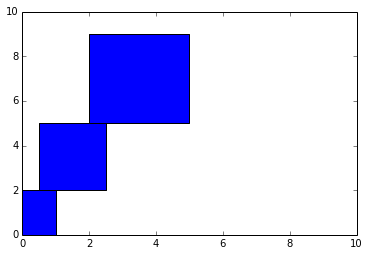Now on to the hard part: the "stacking algorithm".

## Writing a function that computes the stacking¶

How does one write such a stacking function? Answer: this situation is described by static mechanics.

Let's assume we have two blocks: a "top" and a "bottom" block. The horizontal shift for the top block is $x_i$. The forces that apply to the top block are:

• gravity, which is proportional to its mass $m_i$
• the reaction force due to the bottom block (a contact pressure)

In our model, we will assume that the blocks are rigid and thus replace the contact pressure by a point force located at the right most point of the bottom block. This is illustrated by the figure below.

In :
from IPython.display import SVG
SVG(filename='files/forces.svg')

Out:

Under these hypotheses, equilibrium has a simple expression:

• if the center of gravity is located to the left of the pressure force, i.e. the edge of the bottom block, the structure is in a stable state
• if the center of gravity is located to the right of the pressure force, i.e. the edge of the bottom block, the structure is in an unstable state and will collapse

This is illustrated by the figure below:

In :
SVG(filename='files/forces_equilibrium.svg')

Out:

So the algorithm is the following:

• we loop over the bricks to stack in a reverse order, starting with the last brick which goes on top
• we calculate the maximum shift we can apply to the brick at the bottom given the top brick and its mass
• we define a new top brick that consists of the previous top brick plus the previous bottom brick but positioned on top of each other
• we apply the same procedure as above to our new top and bottom bricks

To follow this algorithm, we will need to compute the position of the new center of gravity obtained by assembling bricks together. This is coded below:

In :
def compute_horizontal_center_of_mass(brick_sequence, shifts):
compute_mass = lambda b: b * b
sum_xi_mi = sum([(shifts[i] + brick_sequence[i]/2.) * compute_mass(brick_sequence[i]) for i in range(len(brick_sequence))])
sum_mi = sum([compute_mass(brick_sequence[i]) for i in range(len(brick_sequence))])
return sum_xi_mi / sum_mi


And finally, the algorithm:

In :
def compute_brick_shifts(brick_sequence):
shifts = []
reverse_brick_sequence = brick_sequence[::-1]
for index, brick in enumerate(reverse_brick_sequence):
if index == 0:
shifts.append(0.)
else:
previous_center_of_mass = compute_horizontal_center_of_mass(reverse_brick_sequence[:index], shifts)
shifts.append(previous_center_of_mass - brick)
min_shift = min(shifts)
return [s - min_shift for s in shifts[::-1]]


To test our algorithm, we're going to examine a couple of solutions obtained when stacking rectangular bricks on top of each other. First, we're doing this with an interactive plot:

In :
def plot_nbricks(l, n):
bricks = generate_brick_sequence(l, 1, n)
plot_brick_sequence(bricks, compute_brick_shifts(bricks))
pl.xlim(0, n)
pl.ylim(0, n)
pl.grid(True)

In :
interact(plot_nbricks,
l=(0.1, 4, 0.1),
n=(2, 20))

Out:
<function __main__.plot_nbricks>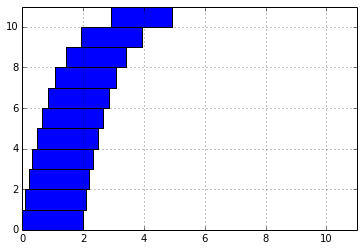Let's check the solution for 10, 20 and 40 bricks of 2:1 aspect ratios:

In :
pl.figure(figsize=(10, 4))
format_plot = lambda : pl.xlim(0, 8) and pl.ylim(0, 40) and pl.grid(True);

pl.subplot(131)
plot_nbricks(2, 4)
format_plot()

pl.subplot(132)
plot_nbricks(2, 13)
format_plot()

pl.subplot(133)
plot_nbricks(2, 40)
format_plot()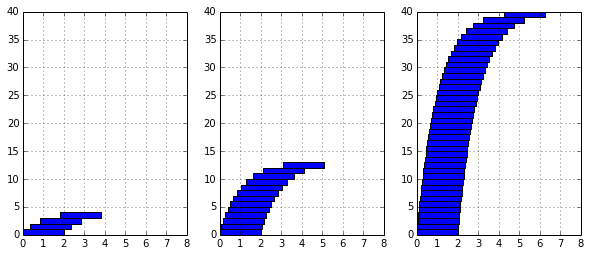As one can see, the more bricks we stack, the higher the tower gets and the larger vaults we can build by simply stacking these bricks on top of each other.

This being said we can now move on to some practical questions.

## Applying our tools to practical questions¶

### How far can we get?¶

One of the questions we can ask ourselves after having written this is: is there a maximum horizontal distance that we can cross with such a structure of stacked bricks? Or is there a limit that we cannot overcome inside our model?

To examine this question, I'll plot the total distance covered as a function of the number of bricks stacked.

In :
l = 1
number_of_bricks = pl.linspace(1, 200, 50, pl.int32)
distances = pl.zeros_like(number_of_bricks)
for index, n in enumerate(number_of_bricks):
bricks = generate_brick_sequence(l, 1, int(n))
shifts = compute_brick_shifts(bricks)
distances[index] = max(shifts) + l/2.

In :
pl.plot(number_of_bricks, distances)
pl.title("distance reached as a function of number of {0} width bricks".format(l))
pl.xlabel('brick unit width (a. u.)')
pl.ylabel('distance covered (a. u.)')
pl.grid(True)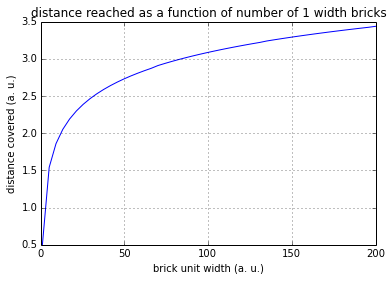This looks like a logarithmic function. And it doesn't look like the growth stops at some point (even though, from this small sampling it looks like it slows down a lot).

If anyone is interested, I found a reference in this book that says the growth is unbounded. Therefore you could build this sort of structure to cover any given distance. In practice, this is limited by the accuracy with which you can place your bricks (and also forces other than gravity...).

### Dependency on the size of the bricks¶

Another question we can ask of our model: how does the range covered by the vault change if we change the brick width?

In :
brick_widths = pl.linspace(0.1, 10)
distances = pl.zeros_like(brick_widths)
for l in brick_widths:
bricks = generate_brick_sequence(l, 1, 100)
distances[brick_widths == l] = max(compute_brick_shifts(bricks)) + l/2.

In :
pl.plot(brick_widths, distances)
pl.xlabel('brick width (distance units)')
pl.ylabel('distance units')
pl.title('distance covered by stacking 100 bricks as a function of brick width')
pl.grid(True)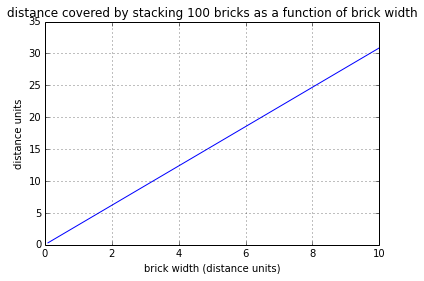The relationship seems linear. We can compute the slope of the previous curve:

In :
(distances[-1] - distances)/(10 - 0.1)

Out:
3.08868875881981

If we increase the brick width by one unit, the we will increase the range of 100 stacked bricks of the same width by 3.088 units.

### Stacking non-uniform sized bricks¶

To finish this post, I'll show how we can stack up arbitrarily shaped bricks. This is one of the nice features of the code we have written so far: it's generic to the point that you can stack bricks of different sizes.

First, we write a function that gives back a sequence of arbitrarily shaped bricks.

In :
def generate_random_brick_sequence(n):
return [(pl.rand(1), 0.2) for i in range(n)]


And then we write a function that just generates a sequence of n bricks, computes the optimal way to stack them and plots them.

In :
def plot_random_bricks(n):
bricks = generate_random_brick_sequence(n)
shifts =  compute_brick_shifts(bricks)
plot_brick_sequence(bricks, shifts)

In :
pl.figure(figsize=(11, 4))
format_plot = lambda : pl.xlim(0, 2.5) and pl.ylim(0, 9) and pl.grid(True);

pl.subplot(131)
plot_random_bricks(40)
format_plot()

pl.subplot(132)
plot_random_bricks(40)
format_plot()

pl.subplot(133)
plot_random_bricks(40)
format_plot()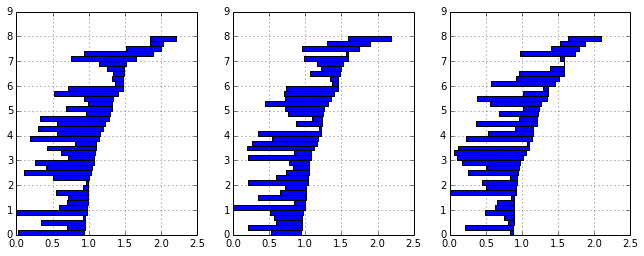# Bonus: an analytical formula for equal sized bricks¶

I first read about the "brick stacking problem" in a physics book called "la physique de tous les jours". The book chapter explains the stacking algorithm that inspired me to write the code in this notebook. In this section, I derive the analytical formula for stacking identical bricks on top of each other. The reasoning is carried out on unit length bricks, but is valid for any size of brick.

In the following, we denote the shift of the left right border of brick $i$ by $s(i)$. The mass of brick of $i$ is written as $m_i$.

The reasoning is the following: suppose you already know the shifts up to order $n$ and you're trying to determine the shift for brick $n+1$. You want the horizontal center of mass of bricks $[1:n]$ to be exactly equal to the shift of brick $n+1$. Thus our first equation:

$$s(n+1) = c_{mass}([1:n])$$

It turns out that the center of mass of the assembly of bricks $[1:n]$ can be computed from the previous shifts. In effect: the center of mass of bricks $[1:n]$ can be written as the center of mass of brick $n$ alone and of bricks $[1:n-1]$. Using the previous equation, we also know that the center of mass of bricks $[1:n-1]$ is equal to the shift $s(n)$. Thus the following formula, derived from the previous relation and weighted by the brick masses:

$$c_{mass}([1:n]) = \frac{(\sum_{i=1}^{n-1} m_i)s(n) + m_n(s(n) + 1/2)}{\sum_{i=1}^{n} m_i}$$

Injecting this formula in our previous equation we obtain a recurrence equation of order 1 on $s(n)$:

$$s(n+1) = \frac{(\sum_{i=1}^{n-1} m_i)s(n) + m_n(s(n) + 1/2)}{\sum_{i=1}^{n} m_i}$$

Assuming that all masses are actually equal, we obtain: $$s(n+1) = \frac{n \, s(n) + 1/2}{n}$$

This relation is to be initialized with $s(1) = 0$.

The following figure illustrates our demonstration:

In :
SVG(filename='files/forces_analytical_formula.svg')

Out:

The formula we derived can easily be coded and compared to what we previously computed.

In :
def analytical_shift(n):
if n==1:
return 0
else:
return 1./(n-1) * ((n-1) * analytical_shift(n-1) + 1/2.)

In :
print [analytical_shift(i) for i in range(1, 10)]

[0, 0.5, 0.75, 0.9166666666666666, 1.0416666666666665, 1.1416666666666664, 1.2249999999999996, 1.296428571428571, 1.358928571428571]

In :
bricks = generate_brick_sequence(1, 1, 10)
shifts = compute_brick_shifts(bricks)
shifts = shifts[::-1]
print [elem - shifts for elem in shifts]

[0.0, -0.5, -0.75, -0.9166666666666666, -1.0416666666666667, -1.1416666666666666, -1.225, -1.2964285714285715, -1.3589285714285715, -1.414484126984127]


Indeed, the results are identical, except for the minus sign that appears in our numerical version.

It turns out that the analytical formula can enable us to prove something we hinted at when examining convergence of a brick stack (subsection "how far can we get?"). We can rewrite the total extent of the arch as:

$$l(n) = \sum_{i=1}^n s(i+1) - s(i) = \frac{1}{2}\sum_{i=1}^n \frac{1}{i}$$

However, as it happens, the series on the right, called the harmonic is divergent. This means that if we stack enough bricks, we can end up with any arch extent we'd like! My physics book tells me that this was first proved by Nicole Oresme, "probably one of the most original thinkers of the 14th century" (to quote Wikipedia).

This post was entirely written using the IPython notebook. You can see a static view or download this notebook with the help of nbviewer at 20140808_BricksAndArches.ipynb.